# Angle of View Calculation in the Basler Lens Selector#

There are several methods to calculate the angle of view of a camera/lens combination, for example:

Method 1: Based on the working distance and the object size: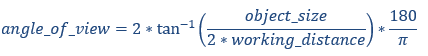Method 2: Based on the lens' focal length and the sensor size: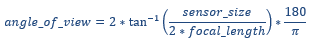The results of these calculations may slightly differ.

The Basler Lens Selector calculates the angle of view with Method 2.

Example: Assume we use a IMX540 camera (a2A-5328) with a 25 mm lens: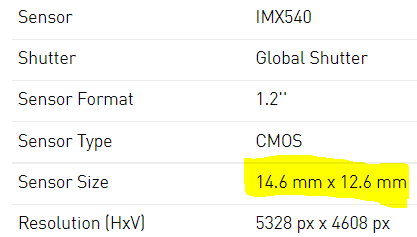Using Method 2, we calculate:And this is exactly what we get in the lens selector: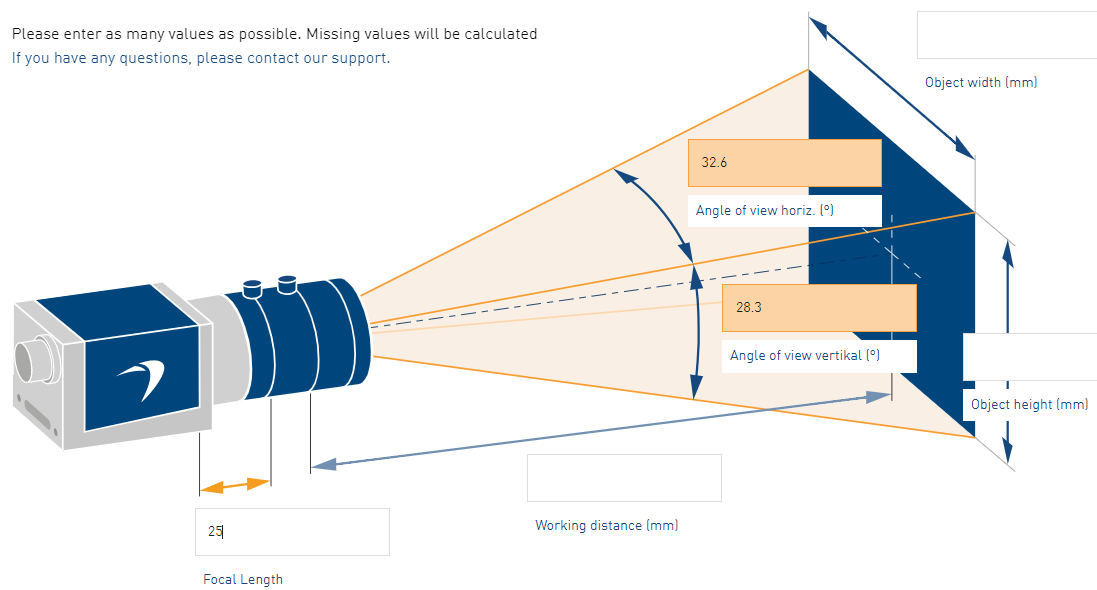If we consider a working distance of 710 mm, the calculator indicates that a focal length of 25 mm will allow viewing an area of 400 mm x 345 mm.However, using Method 1, the angles of view are: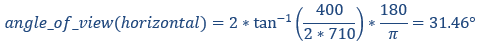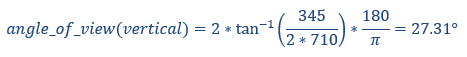You can see that the results are slightly different.

The lens selector tool calculates the angle of view based on the focal length and the sensor size.